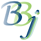# <CORE:Set>

## Description

In BBj 16.0 and higher, the set tag can be used within a BBJSP Page to bind some data to a named attribute in the BBjspPageContext.

## Attributes

Attribute Name

Description

Required

Expression

Data Type

value

the value attribute is the value that should be bound to the name in the BBjspPageContext.

true

true

Object

var

the var attribute is the name to bind to a value in the BBjspPageContext.

true

true

String

## Remarks

The Core Tag Library library needs to be referenced in the BBJSP Page

## Example

 <%-- Import the tag library --%> <%@ taglib uri='/WEB-CFG/tld/core.tld' prefix='c' %> <c:set> <% REM === Make a String s\$ = "A String" REM === Make a BBjVector vect! = new BBjVector() vect!.add("APPLES") vect!.add("BANANAS") vect!.add("ORANGES") vect!.add("GRAPES") salary = 2000 %>
Simple Strings

"" - was set by

"" - was set by

Numerical with calculations Monthly Salary =
Monthly Bonus = - calculated in the expression \${(salary *10 / 100)}
Combined Salary + Bonus = - calculated in the expression \${(salary + bonus)}
Annual Bonus = - calculated in the expression \${(salary * 1.5)}
Annual = - calculated in the expression \${(salary + bonus) *12 + annualBonus}

A BBjVector Vectot Item =